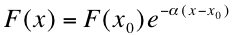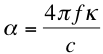Skip to main content

# 1. Absorption Coefficient and Penetration Depth

Light that is transmitted through the semiconductor material is attenuated by a significant amount as it passes through. The rate of absorption of light is proportional to the intensity (the flux of photons) for a given wavelength; in other words, as light passes through the material the flux of photons is diminished by the fact that some are absorbed on the way through. Therefore, the amount of photons that reach a certain point in the semiconductor depends on the wavelength of the photon and the distance from the surface. The following equation models the exponential decay of monochromatic (one-color or approximately single-wavelength) light as it travels through a semiconductor1:where F(x) is the intensity at a point x below the surface of a semiconductor, F(x0) is the intensity at a surface point x0, and α is the absorption coefficient, which determines the depth at which light of a certain wavelength penetrates the semiconductor. The wavelengths most important for solar application are in the infrared and visible parts of the electromagnetic spectrum.

The absorption coefficient is related to the wavelength of light and another quantity called the extinction coefficient, which is also related to the wavelength of light (the electromagnetic waves propagated from the sun). This coefficient κ is an optical property of the semiconductor material and is related to the index of refraction n, which merely determines how much light is absorbed by the material. κ > 0 means absorption, while κ = 0 means the light travels straight through the material. The absorption and extinction coefficients are related by the following equation1:where f is the frequency of the monochromatic light (related to the wavelength by λ=v/ƒ, where v is the velocity of the light wave), c is the speed of light, and π is a constant (≈ 3.14). The absorption coefficient is an important quantity that will show up in the following sections in the various models we have for semiconductor charge carrier generation, so it is good to keep in mind that it depends on both the incoming light and the intrinsic qualities of the material.Above is an image of the ocean and the depth of absorbance by various wavelengths (energies) of light. As you can see, light in the higher and lower energies penetrate the least. In the different case of semiconductors, higher energy photons typically are absorbed more strongly, while low energy photons pass right through the semiconductor.

Source: <disc.sci.gsfc.nasa.gov/educat...ceanblue.shtml>

## References

1. Goetzberger, Adolf et.al. Crystalline Silicon Solar Cells. Chichester: John Wiley & Sons Ltd., 1998.

• Was this article helpful?